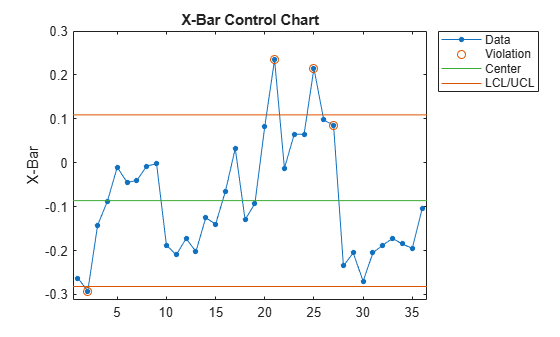# controlrules

Western Electric and Nelson control rules

## Syntax

```R = controlrules('rules',x,cl,se) [R,RULES] = controlrules('rules',x,cl,se) ```

## Description

`R = controlrules('rules',x,cl,se)` determines which points in the vector `x` violate the control rules in `rules`. `cl` is a vector of center-line values. `se` is a vector of standard errors. (Typically, control limits on a control chart are at the values `cl``3*se` and `cl` + `3*se`.) `rules` is the name of a control rule, or a string array or cell array containing multiple control rule names. If `x` has n values and `rules` contains m rules, then `R` is an n-by-m logical array, with `R(i,j)` assigned the value `1` if point `i` violates rule `j`, `0` if it does not.

The following are accepted values for `rules` (specified inside single quotes):

• `we1` — 1 point above `cl` `+` `3*se`

• `we2` — 2 of 3 above `cl` `+` `2*se`

• `we3` — 4 of 5 above `cl` `+` `se`

• `we4` — 8 of 8 above `cl`

• `we5` — 1 below `cl` `–` `3*se`

• `we6` — 2 of 3 below `cl` `–` `2*se`

• `we7` — 4 of 5 below `cl` `–` `se`

• `we8` — 8 of 8 below `cl`

• `we9` — 15 of 15 between `cl` `–` `se` and `cl` `+` `se`

• `we10` — 8 of 8 below `cl` `–` `se` or above `cl` `+` `se`

• `n1` — 1 point below `cl` `–` `3*se` or above `cl` `+` `3*se`

• `n2` — 9 of 9 on the same side of `cl`

• `n3` — 6 of 6 increasing or decreasing

• `n4` — 14 alternating up/down

• `n5` — 2 of 3 below `cl` `–` `2*se` or above `cl` `+` `2*se`, same side

• `n6` — 4 of 5 below `cl` `–` `se` or above `cl` `+` `se`, same side

• `n7` — 15 of 15 between `cl` `–` `se` and `cl` `+` `se`

• `n8` — 8 of 8 below `cl` `–` `se` or above `cl` `+` `se`, either side

• `we` — All Western Electric rules

• `n` — All Nelson rules

For multi-point rules, a rule violation at point `i` indicates that the set of points ending at point `i` triggered the rule. Point `i` is considered to have violated the rule only if it is one of the points violating the rule's condition.

Any points with `NaN` as their `x`, `cl`, or `se` values are not considered to have violated rules, and are not counted in the rules for other points.

Control rules can be specified in the `controlchart` function as values for the `'rules'` parameter.

`[R,RULES] = controlrules('rules',x,cl,se)` returns a cell array of text `RULES` listing the rules applied.

## Examples

collapse all

`load parts;`

Create an Xbar chart using the `we2` rule to mark out of control measurements.

```st = controlchart(runout,'rules','we2'); x = st.mean; cl = st.mu; se = st.sigma./sqrt(st.n); hold on plot(cl+2*se,'m')```You can see the out of control points marked with a red circle.

Use `controlrules` to identify the measurements that violate the control rule.

```R = controlrules('we2',x,cl,se); I = find(R)```
```I = 6×1 21 23 24 25 26 27 ```